International Journal of Economic Behavior and Organization
Volume 3, Issue 2-1, April 2015, Pages: 73-76

A Monetarist Model Reconsidered: The Emergence of Chaotic Fluctuations

Hiroyuki Yoshida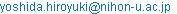Hiroyuki Yoshida. A Monetarist Model Reconsidered: The Emergence of Chaotic Fluctuations. International Journal of Economic Behavior and Organization. Special Issue: Recent Developments of Economic Theory and Its Applications. Vol. 3, No. 2-1, 2015, pp. 73-76. doi: 10.11648/j.ijebo.s.2015030201.21

Abstract: Friedman (1968) proposes a constant money growth rate rule to establish the stability of the market economy. We examine whether his suggestion is reasonable or not. We seek to develop the Vanderkamp (1975) model, which captures the essential points of monetarism: the quantity theory of money and the natural rate of unemployment. Our main finding is that the economy experiences chaotic fluctuations around the steady state when the adjustment speed of inflationary expectations is sufficiently slow.

Keywords: Monetarist, Constant Money Growth Rate Rule, Chaotic Fluctuations

Contents

1. Introduction

Milton Friedman is a leading advocate of monetarism. He has full confidence in the inherent stability of the market economy; namely, the economy tends to return to the long-run steady state automatically without government intervention. As a natural consequence, he has an aversion to discretionary policy to stabilize the economy.

Furthermore, he emphasizes that monetary disturbances are a main source of economic fluctuations. This topic is studied by Friedman and Schwartz (1963) from an empirical point of view. What is more, he expresses the role of monetary policy in his presidential address to the American Economic Association. He declares that a constant money growth rate rule is preferable to discretionary monetary policy. On this point, see Friedman (1968).

The purpose of the present paper is to examine the monetarist doctrine within the theoretical framework. In particular, we examine whether a constant money growth rate rule guarantees the stability of the market economy. This paper seeks to develop the Vanderkamp (1975) model, which captures the essential points of monetarism: the quantity theory of money and the natural rate of unemployment. Vanderkamp, however, does not introduce explicitly a mathematical formulation of inflationary expectations into his model. For this reason, his analysis is achieved by a graphical method alone. In contrast, we shall advance our analysis by incorporating the adaptive expectations formula in our model.

We focus our attention on the possibility of persistent fluctuations in the key macroeconomic variables such as the unemployment rate and the inflation rate. For this purpose we use the theory of nonlinear dynamical systems, which is a powerful and useful tool to identify endogenous cycles. From an analytical point of view, we show the birth of a period-two cycle via a flip bifurcation. Moreover, we explore the emergence of chaotic fluctuations by means of numerical simulations: we observe a period-doubling route to chaos through a bifurcation diagram.

This paper is organized as follows. Section 2 explains the basic framework. In Section 3 we investigate the dynamic properties of the model. Section 4 presents some numerical results of our computer calculations. Section 5 summarizes our results.

2. The Basic Framework

The following model is a discrete-time version of the Vanderkamp (1975) model, which is a prototype monetarist model.

We consider the following equations of the model: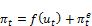(1)(2)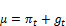(3)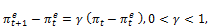(4)

where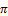is the rate of inflation,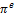is the expected rate of inflation,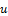represents the rate of unemployment, and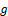is the growth rate of output. They are endogenous variables and all functions of time. In addition, the model includes the following exogenous parameters: the natural growth rate,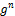, the growth rate of nominal money supply,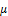, and the adjustment speeds,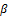andEquation (1) is the expectations-augmented version of the price-Phillips curve. The derivation is as follows. First, it is well known that Phillips (1958) finds an inverse relationship between the rate of unemployment and the rate of increase of money wages. The original Phillips curve is represented as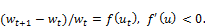(5)

The function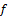shows that the lower the rate of unemployment, the higher the rate of money-wage inflation and vice versa.

Later, Friedman (1968) and Phelps (1968) emphasize the importance of inflationary expectations in the money-wage dynamics. Their idea establishes the expectations-augmented Phillips curve: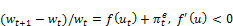(6)

Furthermore, we assume that firms maintain a constant mark-up of prices over unit labor costs. Then we obtain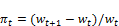(7)

It is obvious from (6) and (7) that we can derive the expectations-augmented version of the price-Phillips curve (1).

Following Vanderkamp (1975, p.118), we introduce (2) as an "equilibrating mechanism" in our model. It is a kind of Okun's law, which describes a relationship between the "growth rate" of the unemployment rate and the growth rate of output. In most cases, Okun's law is formulated as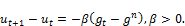(8)

This standard specification represents a linear relationship between the "change" in the unemployment rate and the growth rate of output. Broadly speaking, both specifications convey that the unemployment rate decreases when the growth rate of output exceeds the natural growth rate. Nevertheless, (2) is different from (8) in that our formulation brings about an intrinsic nonlinearity to generate complex dynamics in our model.

Equation (3) shows the market clearing condition for the money market. It is assumed that the demand for moneyis proportional to the nominal level of output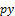, that is,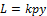, where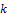is a constant multiplier,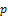is the price level, andis the real level of output. On the other hand, we assume that the central bank maintains a constant growth rate of nominal money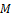: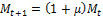. Equilibrium in the money market requires that money supply be equal to money demand. Using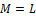, we can obtain (3).

Equation (4) explains the revision of inflationary expectations. In the present paper we assume the adaptive expectations scheme: inflationary expectations are revised in proportion to the forecast error. As discussed in Introduction, we reinforce the Vanderkamp model by introducing a mathematical specification of inflationary expectations.

Before closing this section, it is worth mentioning the related papers. First, the papers by Soliman (1996a, 1996b) investigate simple inflation-unemployment models, which are similar to our model. His papers show the emergence of chaos by using nonlinear discrete dynamic systems. However, unlike our model, he constructs his models without considering Okun's law. The point to observe is that the major source of nonlinearity is the Phillips curve in his models.

Secondly, Lines and Westerhoff (2010) also study a model of inflation and unemployment by using a simple macroeconomic framework. They focus attention on the heterogeneous expectation formation and show the occurrence of complex dynamics.

3. Dynamic Analysis

In this section we investigate the dynamic properties of a simple monetarist model. The complete dynamical system can be derived from (1), (2), (3), and (4).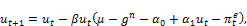(9a)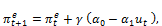(9b)

where we specify the Phillips curve as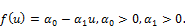(10)

At the steady state,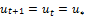and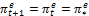. From (9) we recognize that there is a unique steady state in our system: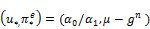.

This result indicates two important features in the long-run situation. First, the long-run Phillips curve is vertical at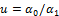. It is therefore appropriate to conclude that the natural rate of unemployment is equal to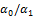in this model. Secondly, the neutrality of money holds in the long run. A permanent change in the growth rate of money supply has no impact on real variables such as the economic growth rate and the unemployment rate in the long run.

The Jacobi matrix evaluated at the steady state is given by(11)

Then we can obtain the corresponding characteristic equation:(12)

To determine the local stability of the steady state, we have to check the Schur-Cohn criterion, which provides a necessary and sufficient condition for dynamic stability.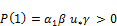(13a)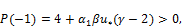(13b)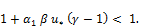(13c)

It is easy to verify that (13a) and (13c) are always satisfied since our analysis is restricted to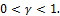Thus, rearranging (13b), we obtain the local stability condition.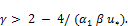(14)

This implies that the local stability of the steady state is ensured if the adjustment speed of inflationary expectations is sufficiently fast. On the other hand, rearranging (13b) again, we get(15)

This means that the local stability is violated if the adjustment speed of the equilibrium mechanism via Okun's law is sufficiently fast.

There is one other thing that is important; the violation of (13b) is associated with a flip bifurcation, which appears when one eigenvalue is equal to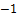. In this case the system undergoes a flip bifurcation when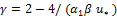.

4. Numerical Results

From the analytical point of view, we have investigated the local stability condition for the monetarist model in the preceding section. In this section we shall carry out the numerical simulation analysis of the monetarist model. The analysis is useful and important in studying the global dynamics. See, for example, Devaney (2003) and Guckenheimer and Holmes (2002) for a detailed presentation of chaotic dynamical systems.

The following values for the exogenous parameters are chosen: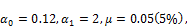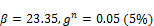.

In the above setting we have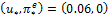. In addition to this, we can calculate a flip bifurcation value:That is, the steady state is locally stable for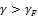, and unstable for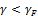.

Figures 1-4 show a period-doubling route to chaos, which is a typical phenomenon in chaotic dynamics. These figures depict the time paths of our model in the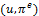-plane. In general, an infinite number of period-doubling bifurcations leads to chaos. Such a route to chaos is called the Feigenbaum scenario.

Figure 1 is obtained by fixing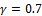. Note that we report the final trajectory by removing transient trajectories of the model. Thus, we observe only the steady state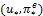,  which is unique and stable in this system.

Figure 2 displays a stable cycle of period 2. Decreasing the value offrom 0.7 to 0.55, the system undergoes a flip bifurcation. This is because, as has been pointed out, the flip bifurcation valueis 0.572.

A further decrease to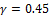causes a stable cycle of period 4 through a flip bifurcation. This result is depicted in Figure 3. Although we omit the detailed results of our analysis, we can observe the occurrence of further period-doubling bifurcations, giving cycles of period 8 and period 16.

By setting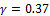, we provide Figure 4. In this case we can see a strange attractor with chaotic fluctuations around the steady state.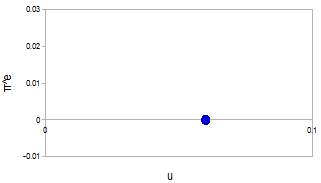Figure 1. Stable steady state,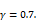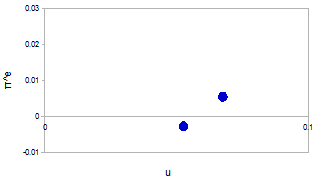Figure 2. Cycle of period 2,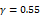.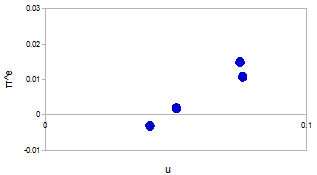Figure 3. Cycle of period 4,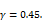Figure 4. Chaotic attractor,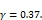We have so far confirmed the occurrence of the period-doubling phenomenon by observing a few phase diagrams.  Finally, we depict a bifurcation diagram forranging from 0.37 to 0.65, as in Figure 5. The diagram plots all possible values of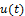in the final trajectories as a function of the adjustment speed of inflationary expectations. It shows clearly that a cascade of successive period-doubling bifurcations occurs when changing the value of. This result implies that chaotic motions appear in this system.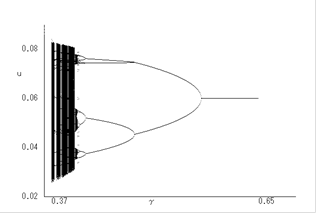Figure 5. The bifurcation diagram with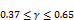.

5. Summary

We have investigated the discrete-time version of the Vanderkamp (1975) model by using nonlinear dynamics. The model is constructed on the basis of the quantity theory of money, the expectations-augmented Phillips curve, and Okun's law.

From what has been discussed above, we can conclude two main points. The first point is that our model accepts the neutrality of money and the natural rate of unemployment hypothesis in the long-run situation.

The second point is that the steady state of the economy might be unstable under the constant money growth rate rule. If the adjustment speed of inflationary expectations is sufficiently slow, then the economy experiences chaotic fluctuations around the steady state. In consequence, we should notice that the central bank cannot always attain the stability of the market economy by using the constant money growth rate rule.

Acknowledgements

The author is deeply grateful to an anonymous referee for useful comments and suggestions. This paper was presented at the 62nd Annual Conference of the Japan Society of Political Economy, October, 2014. The author thanks Kazuhiro Kurose, Takashi Ohno, Hiroaki Sasaki, Masanori Yokoo, and Naoki Yoshihara for helpful comments. All errors are the sole responsibility of the author. Finally and most importantly, this paper is dedicated to Professor Toshikazu Ito on the occasion of his retirement from Ryukoku University.

References

1. Devaney, R. L. (2003) An Introduction to Chaotic Dynamical Systems. Colorado: Westview Press.
2. Friedman, M. (1968) The role of monetary policy. American Economic Review, 58, 1-17.
3. Friedman, M. and Schwartz, A. J. (1963) A Monetary History of the United States, 1867-1960. Princeton: Princeton University Press.
4. Guckenheimer, J. and Holmes, P. (2002) Nonlinear Oscillations, Dynamical Systems, and Bifurcations of Vector Fields. Corrected 6th Printing. Berlin: Springer.-Verlag.
5. Phelps, E. S. (1968) Money-wage dynamics and labor-market equilibrium. Journal of Political Economy, 76, 678-711.
6. Phillips, A. W. (1958) The relation between unemployment and the rate of change of money wage rates in the United Kingdom, 1861-1957. Economica, 25, 283-299.
7. Soliman, A. S. (1996a) Fractals in nonlinear economic dynamic systems. Chaos, Solitons & Fractals, 7, 247-256.
8. Soliman, A. S. (1996b) Transitions from stable equilibrium points to periodic cycles to chaos in a Phillips curve system. Journal of Macroeconomics, 18, 139-153.
9. Lines, M. and Westerhoff, F. (2010) Inflation expectations and macroeconomic dynamics: The case of rational versus extrapolative expectations. Journal of Economic Dynamics and Control, 34, 246-257.
10. Vanderkamp, J. (1975) Inflation: A simple Friedman theory with a Phillips twist. Journal of Monetary Economics, 1, 117-122.

Article ToolsAbstractPDF(249K)